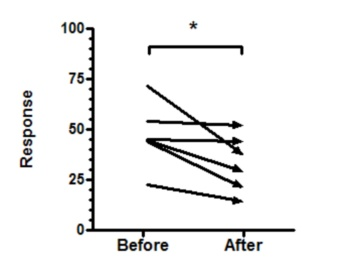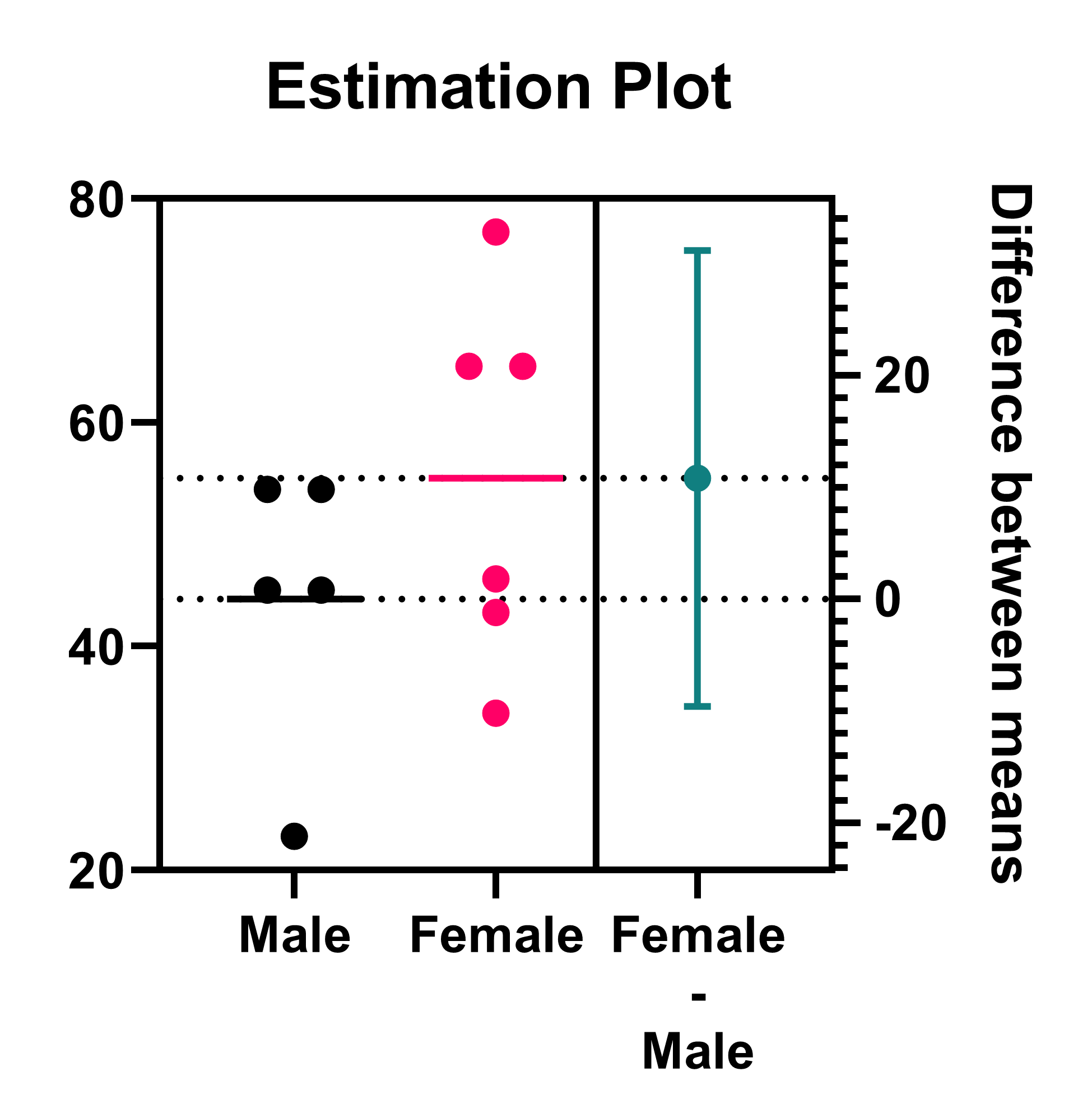## Please enable JavaScript to view this site.

 Graphing tips: Unpaired t

## Points or bars?The graphs above plot the sample data for an unpaired t test. We prefer the graph on the left which shows each individual data point. This shows more detail, and is easier to interpret, than the bar graph on the right.

## Graphing tips

The scatter plot shows a horizontal line at the mean. If you choose the nonparametric Mann-Whitney test, you'll probably want to plot the median instead (a choice in the Format Graph dialog). Prism lets you turn off the horizontal line altogether.

The horizontal line with caps is easy to draw. Draw a line using the tool in the Draw section of the toolbar. Then double click that line to bring up the Format Object dialog, where you can add the caps.

The text objects "P=" and "95% CI of Difference" were created separately than the values pasted from the results. Click the text "T" button, then click on the graph and type the text.

Don't forget to state somewhere how the error bars are calculated. We recommend plotting the mean and SD if you analyze with an unpaired t test, and the median and Interquartile range if you use the nonparametric Mann-Whitney test.

If you choose a bar graph, don't use a log scale on the Y axis. The whole point of a bar graph is that viewers can compare the height of the bars. If the scale is linear (ordinary), the relative height of the bars is the same as the ratio of values measured in the two groups. If one bar is twice the height of the other, its value is twice as high. If the axis is logarithmic, this relationship does not hold. If your data doesn't show well on a linear axis, either show a table with the values, or plot a graph with individual symbols for each data point (which work fine with a log axis).

For the same reason, make sure the axis starts at Y=0 and has no discontinuities. The whole idea of a bar graph is to compare height of bars, so don't do anything that destroys the relationship between bar height and value.

## Including results on the graph

You can copy and paste any results from the results table onto the graph. The resulting embedded table is linked to the results. If you edit the data, Prism will automatically recalculate the results and update the portions pasted on the graph.

The graph on the left shows the exact P value. The graph on the right just shows the summary of significance ("ns" in this case, but one or more asterisks with different data). I recommend you show the exact P value. This summary can be added automatically by using the Pairwise Comparisons button in the Draw section of the toolbar.The most useful information from an unpaired t test is the difference between the two means, and the confidence interval for this difference. This confidence interval is pasted onto the graph above on the left. However, using an Estimation plot (created by default for unpaired t tests) is a much more informative way to display this information.